Ex 9.4

Chapter 9 Class 12 Differential Equations
Serial order wise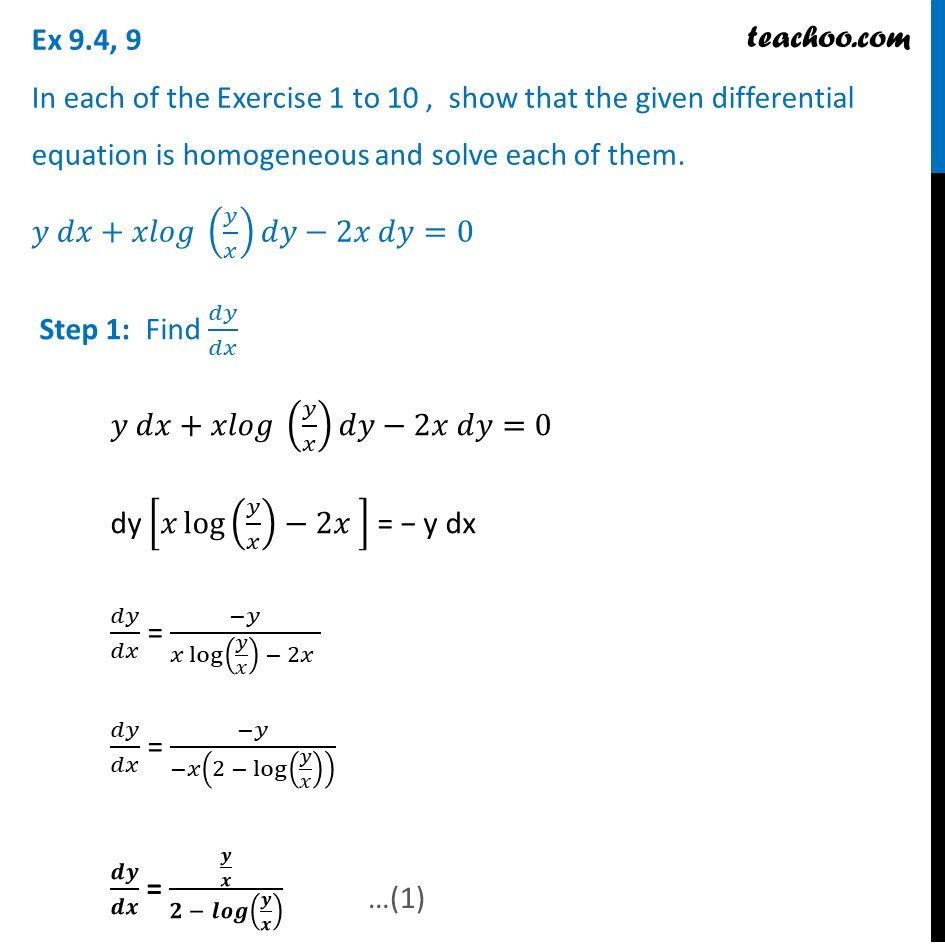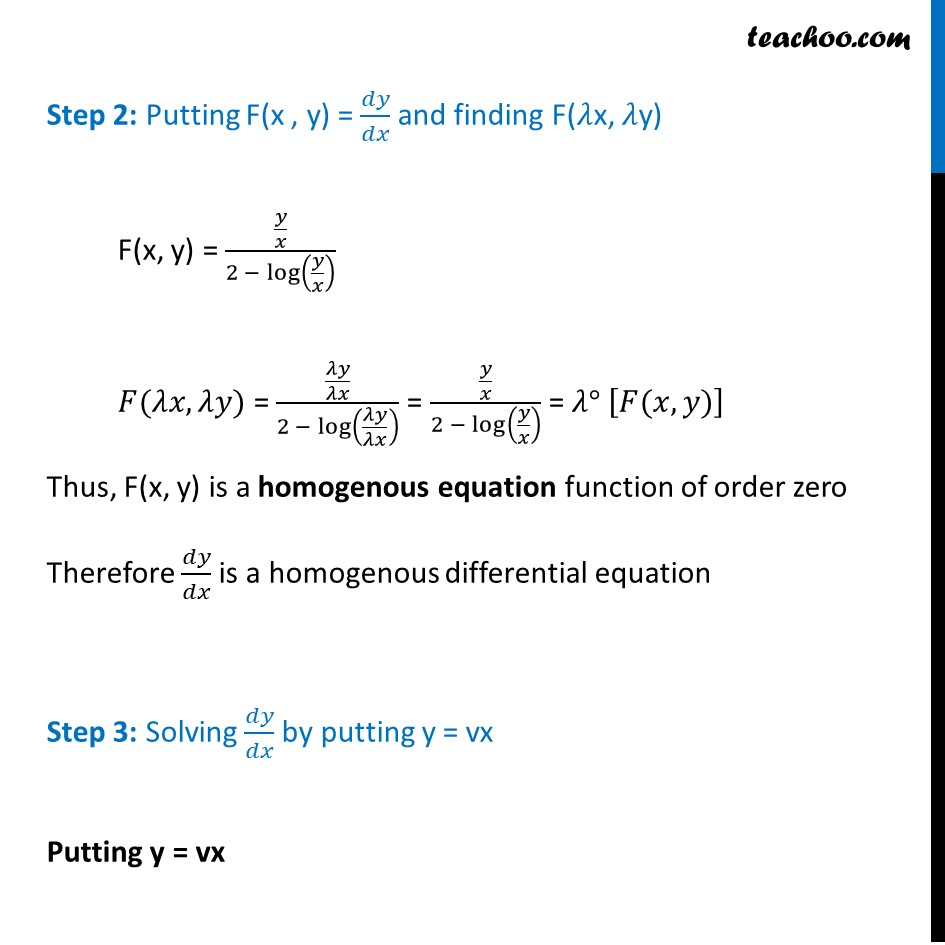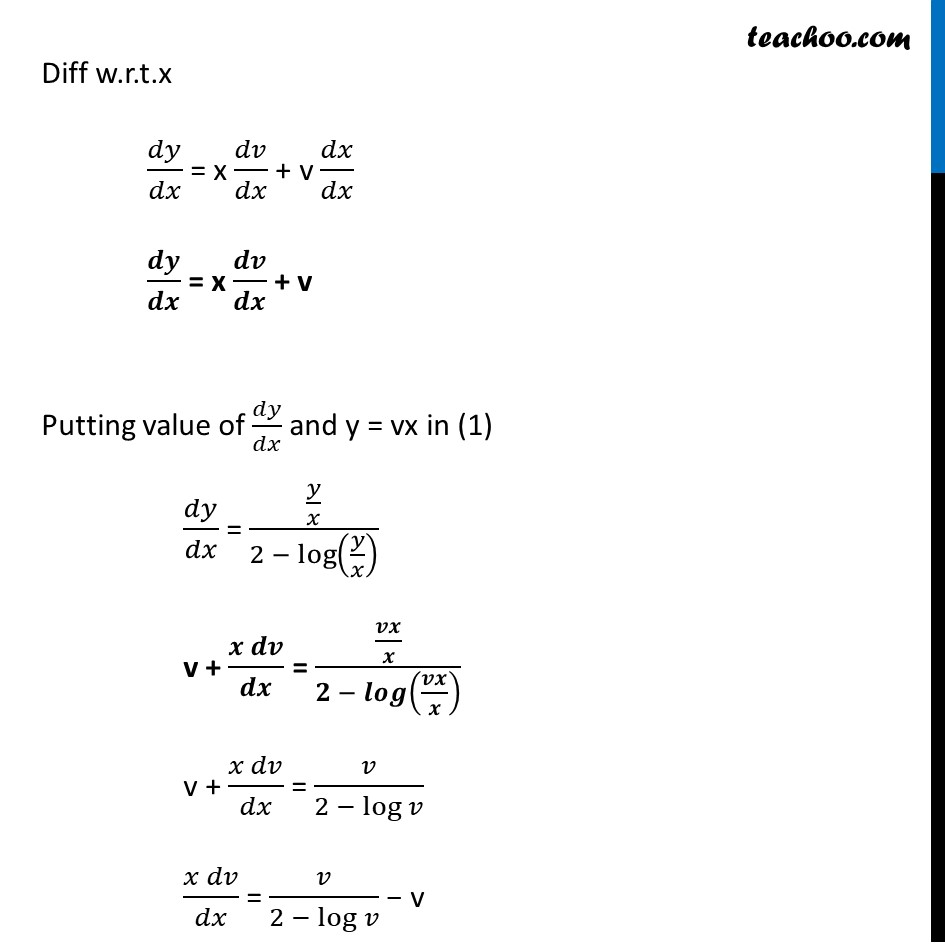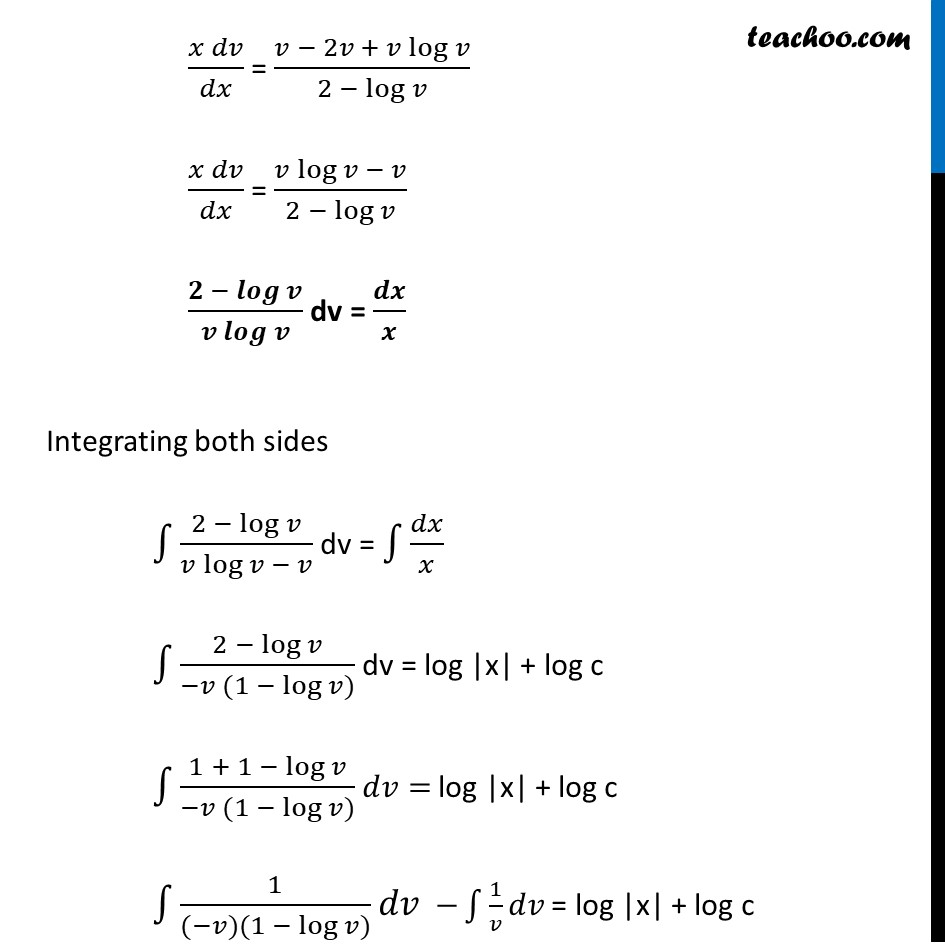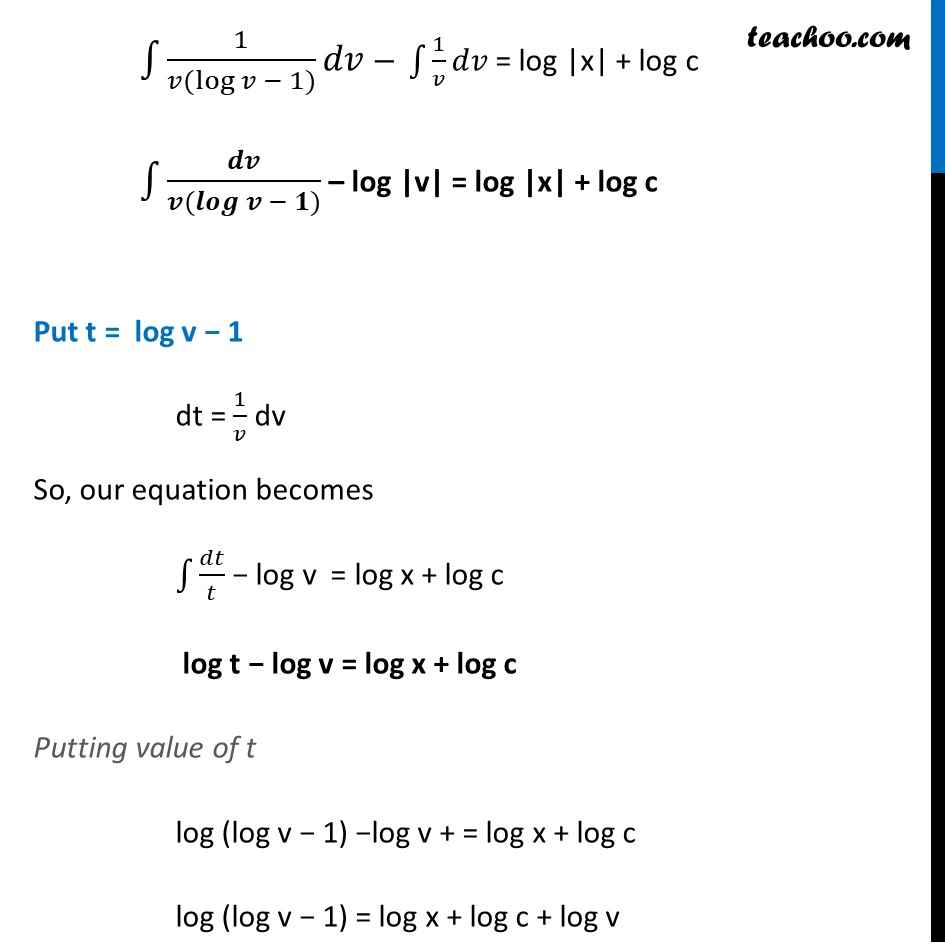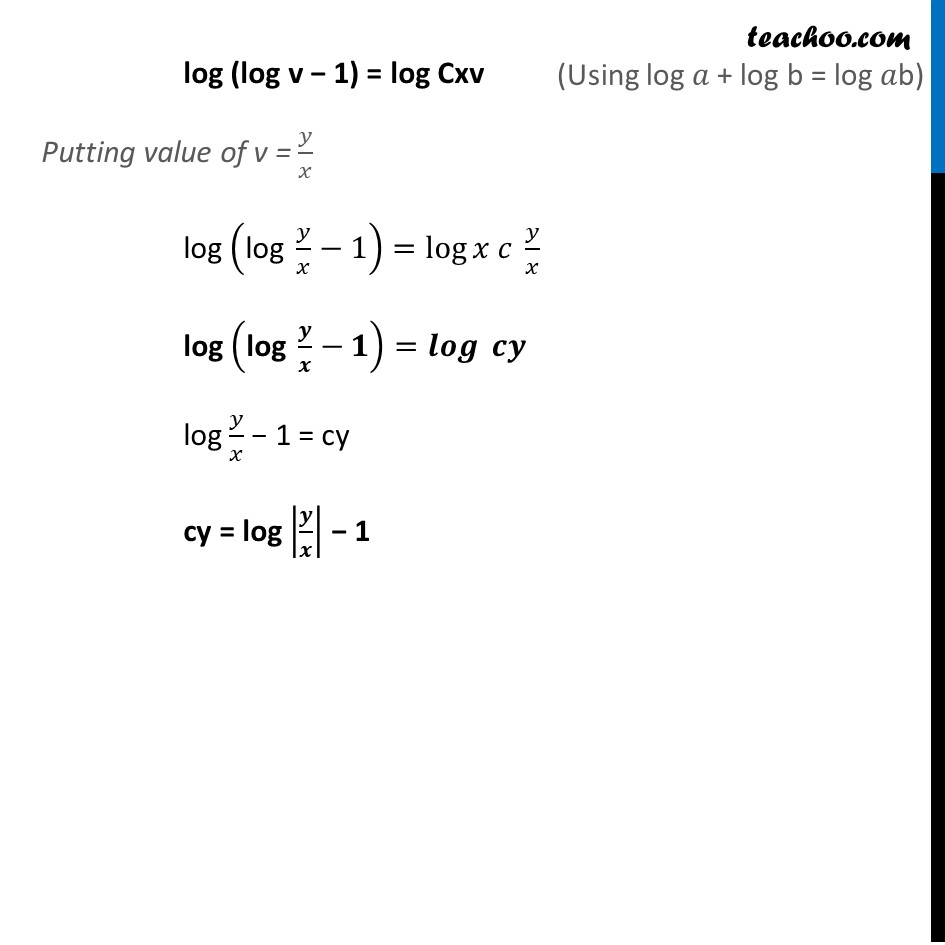Learn in your speed, with individual attention - Teachoo Maths 1-on-1 Class

### Transcript

Ex 9.4, 9 In each of the Exercise 1 to 10, show that the given differential equation is homogeneous and solve each of them. 𝑦 𝑑𝑥+𝑥𝑙𝑜𝑔 (𝑦/𝑥)𝑑𝑦−2𝑥 𝑑𝑦=0 Step 1: Find 𝑑𝑦/𝑑𝑥 𝑦 𝑑𝑥+𝑥𝑙𝑜𝑔 (𝑦/𝑥)𝑑𝑦−2𝑥 𝑑𝑦=0 dy [𝑥 log⁡〖(𝑦/𝑥)−2𝑥 〗 ] = − y dx 𝑑𝑦/𝑑𝑥 = (−𝑦)/(𝑥 log⁡〖(𝑦/𝑥) − 2𝑥 〗 ) 𝑑𝑦/𝑑𝑥 = (−𝑦)/(−𝑥(2 − log⁡(𝑦/𝑥) ) ) 𝒅𝒚/𝒅𝒙 = (𝒚/𝒙)/(𝟐 − 𝒍𝒐𝒈⁡(𝒚/𝒙) ) Step 2: Putting F(x , y) = 𝑑𝑦/𝑑𝑥 and finding F(𝜆x, 𝜆y) F(x, y) = (𝑦/𝑥)/(2 − log⁡(𝑦/𝑥) ) 𝐹(𝜆𝑥,𝜆𝑦) = (𝜆𝑦/𝜆𝑥)/(2 − log⁡(𝜆𝑦/𝜆𝑥) ) = (𝑦/𝑥)/(2 − log⁡(𝑦/𝑥) ) = 𝜆° [𝐹(𝑥, 𝑦)] Thus, F(x, y) is a homogenous equation function of order zero Therefore 𝑑𝑦/𝑑𝑥 is a homogenous differential equation Step 3: Solving 𝑑𝑦/𝑑𝑥 by putting y = vx Putting y = vx Diff w.r.t.x 𝑑𝑦/𝑑𝑥 = x 𝑑𝑣/𝑑𝑥 + v 𝑑𝑥/𝑑𝑥 𝒅𝒚/𝒅𝒙 = x 𝒅𝒗/𝒅𝒙 + v Putting value of 𝑑𝑦/𝑑𝑥 and y = vx in (1) 𝑑𝑦/𝑑𝑥 = (𝑦/𝑥)/(2 − log⁡(𝑦/𝑥) ) v + (𝒙 𝒅𝒗)/𝒅𝒙 = (𝒗𝒙/𝒙)/(𝟐 − 𝒍𝒐𝒈(𝒗𝒙/𝒙) ) v + (𝑥 𝑑𝑣)/𝑑𝑥 = 𝑣/(2 − log⁡𝑣 ) (𝑥 𝑑𝑣)/𝑑𝑥 = 𝑣/(2 − log⁡𝑣 ) − v (𝑥 𝑑𝑣)/𝑑𝑥 = (𝑣 − 2𝑣 + 𝑣 log⁡𝑣)/(2 − log⁡𝑣 ) (𝑥 𝑑𝑣)/𝑑𝑥 = (𝑣 log⁡𝑣 − 𝑣)/(2 −〖 log〗⁡𝑣 ) (𝟐 − 𝒍𝒐𝒈⁡𝒗)/(𝒗 𝒍𝒐𝒈⁡𝒗 ) dv = 𝒅𝒙/𝒙 Integrating both sides ∫1▒(2 − log⁡𝑣)/(𝑣 log⁡𝑣 − 𝑣) dv = ∫1▒𝑑𝑥/𝑥 ∫1▒(2 − log⁡𝑣)/(−𝑣 〖(1 − log〗⁡𝑣)) dv = log |x| + log c ∫1▒(1 + 1 − log⁡𝑣)/(−𝑣 〖(1 − log〗⁡𝑣)) 𝑑𝑣= log |x| + log c ∫1▒1/((−𝑣)(1 − log⁡〖𝑣)〗 ) 𝑑𝑣 −∫1▒1/𝑣 𝑑𝑣 = log |x| + log c ∫1▒1/(𝑣(log⁡𝑣 − 1)) 𝑑𝑣 − ∫1▒1/𝑣 𝑑𝑣 = log |x| + log c ∫1▒𝒅𝒗/(𝒗(𝒍𝒐𝒈⁡〖𝒗 − 𝟏)〗 ) – log |v| = log |x| + log c Put t = log v − 1 dt = 1/𝑣 dv So, our equation becomes ∫1▒𝑑𝑡/𝑡 − log v = log x + log c log t − log v = log x + log c Putting value of t log (log v − 1) −log v + = log x + log c log (log v − 1) = log x + log c + log v log (log v − 1) = log Cxv Putting value of v = 𝑦/𝑥 log ("log " 𝑦/𝑥−1)=log⁡〖𝑥 𝑐 𝑦/𝑥〗 log ("log " 𝒚/𝒙−𝟏)=𝒍𝒐𝒈⁡〖 𝒄𝒚〗 log 𝑦/𝑥 − 1 = cy cy = log |𝒚/𝒙| − 1